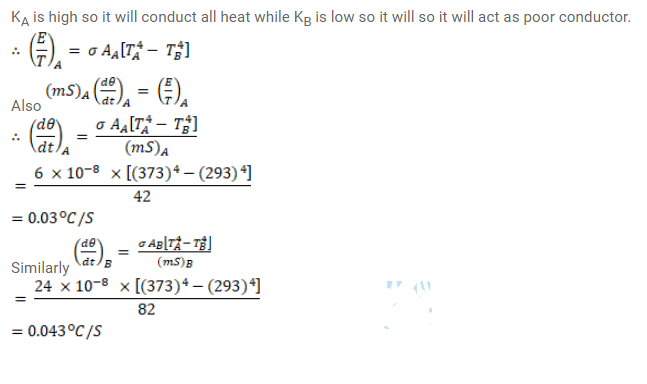# A spherical ball A of surface area 20cm squer is kept at the centre ofQuestion:

A spherical ball A of surface area $20 \mathrm{~cm}^{2}$ is kept at the centre of a hollow spherical shell B of area $80 \mathrm{~cm}^{2}$. The surface of $A$ and the inner surface of $B$ emit as blackbodies. Assume that the thermal conductivity of the material of $B$ is very poor and that of $A$ is very high and that the air between $A$ and $B$ has been pumped out. The heat capacities of $\mathrm{A}$ and $\mathrm{B}$ are $42 \mathrm{~J} /{ }^{\circ} \mathrm{C}$ and $82 \mathrm{~J} /{ }^{\circ} \mathrm{C}$ respectively. Initially, the temperature of $A$ is $100^{\circ} \mathrm{C}$ and that of $B$ is $20^{\circ} \mathrm{C}$. Find the rate of change of temperature of $A$ and that of $B$ at this instant. Explain the effects of the assumptions listed in the problem.

Solution: